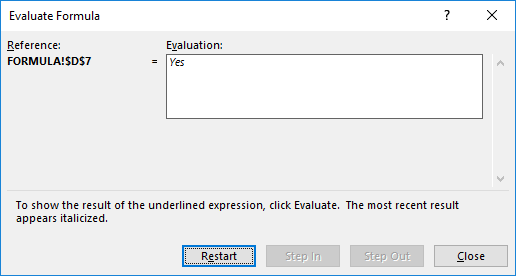This is one of the coolest tricks I have seen in Excel, as there are countless times wherein I had a hard time understand formulas. Especially long and complex ones!

Excel provides the way to evaluate your formula, and break it down step by step so that you can understand it!

Let us take the formulas I’ve created below in the IS THE VALUE IN BETWEEN column . We will see how this formula is resolved in a series of steps: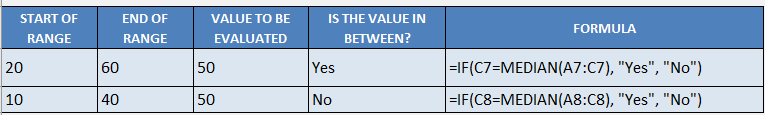STEP 1: You can see our formula uses both the If formula and the Median formula.

The goal of this formula is to evaluate if a value (VALUE TO BE EVALUATED) is in between the range (START OF RANGE to VALUE TO BE EVALUATED)

For example: Is 50 the median of the range 20; 60; 50?

## =IF(C7=MEDIAN(A7:C7), “Yes”, “No”)

To start understanding our formula, highlight the formula, then go to Formulas > Evaluate Formula: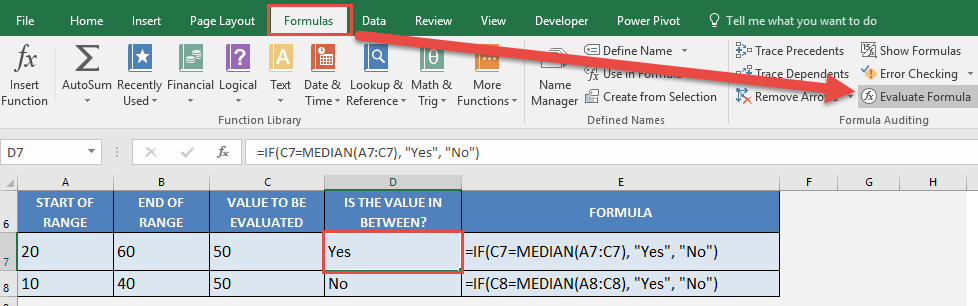STEP 2: Our formula is now shown on screen, and the part that is underlined is the one to be evaluated first.  Click Evaluate.

##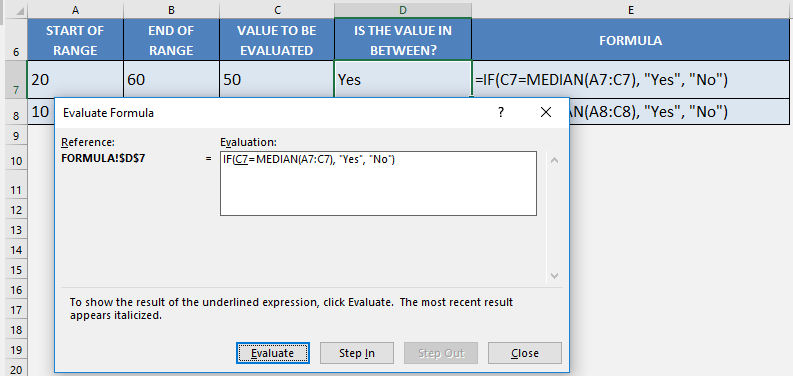STEP 3: C7 has been evaluated to 50.  Click Evaluate.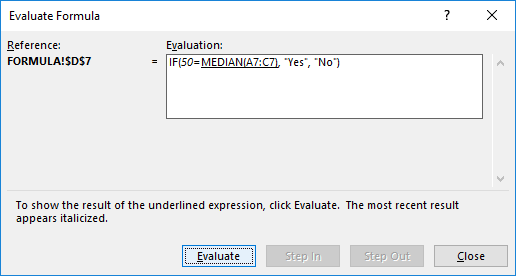STEP 4:  The median of the values from A7 to C7 (20, 60, 50) is evaluated as 50.  Click Evaluate.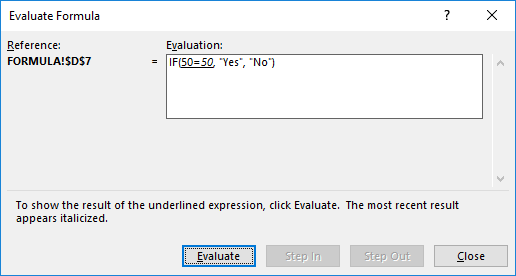STEP 5:  Is 50 equal to 50?

Excel has evaluated it to TRUE.  Click Evaluate.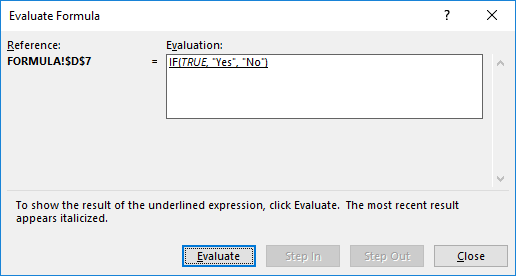STEP 6:  Since the If formula received a TRUE, Excel evaluated it as a Yes end result. We have seen how the formula gave us the result in a few easy steps!